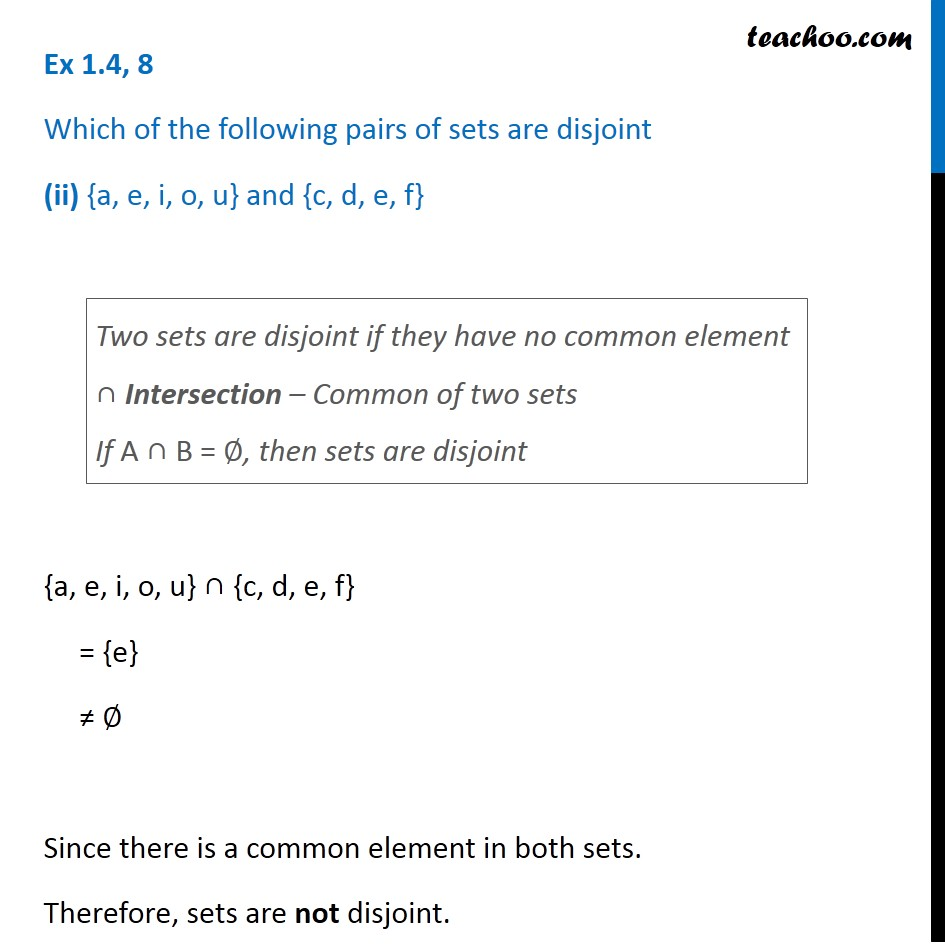Ex 1.4

Chapter 1 Class 11 Sets
Serial order wiseLearn in your speed, with individual attention - Teachoo Maths 1-on-1 Class

### Transcript

Ex 1.4, 8 Which of the following pairs of sets are disjoint (ii) {a, e, i, o, u} and {c, d, e, f} {a, e, i, o, u} ∩ {c, d, e, f} = {e} ≠ ∅ Since there is a common element in both sets. Therefore, sets are not disjoint. Two sets are disjoint if they have no common element ∩ Intersection – Common of two sets If A ∩ B = ∅, then sets are disjoint